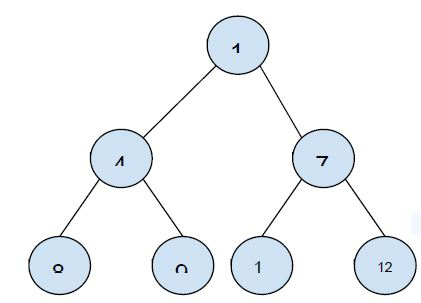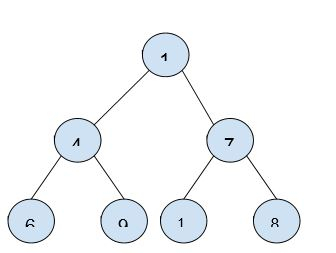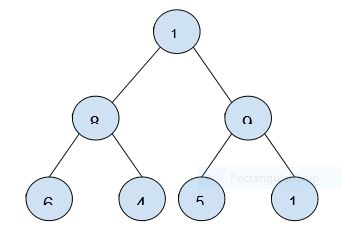# Array Representation Of Binary Heap

CServer Side ProgrammingProgramming

The complete binary tree that follows the properties of heap ordering is called binary heap.

Based on the ordering of binary heap, it can be of two types −

min Heap is the heap in which the value of node is greater than or equal to the value of its parent node. The root node of min heap is smallest.

max Heap is the heap in which the value of node is smaller than or equal to the value of its parent node. The root node of max heap is greatest.

The values of binary heap is typically represented as an array. The array representation of binary heap as −

• Index of the root element is 0.

• If i is the index of the node in the array. Then, the other nodes related to the node are index in the array as −

• Left child : (2*i)+1

• Right child : (2*i)+2

• Parent child : (i-1)/2

Using the above rules of array representation of we can represent a heap in array −1 4 7 8 9 11 12

Now, the types of heaps based on ordering can be discussed here

• Min Heap − The root node has the minimum value. The value of node is greater than value of parent node.

Example −Array representation −

 1 4 7 6 9 10 8
•  Max Heap − The root node has the maximum node. The value of node is smaller than the value of parent node.

Example −Array representation −

 11 8 9 6 4 5 1